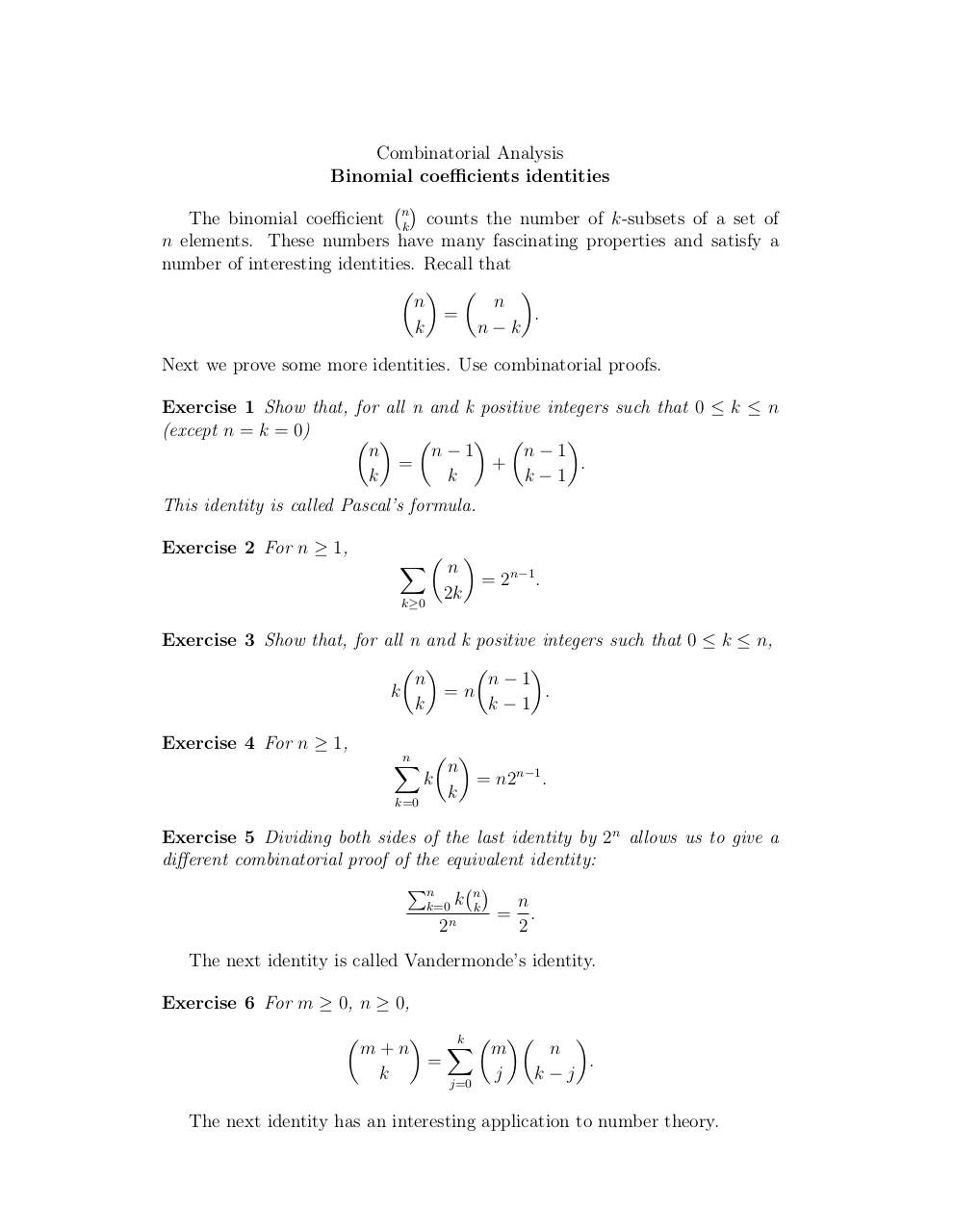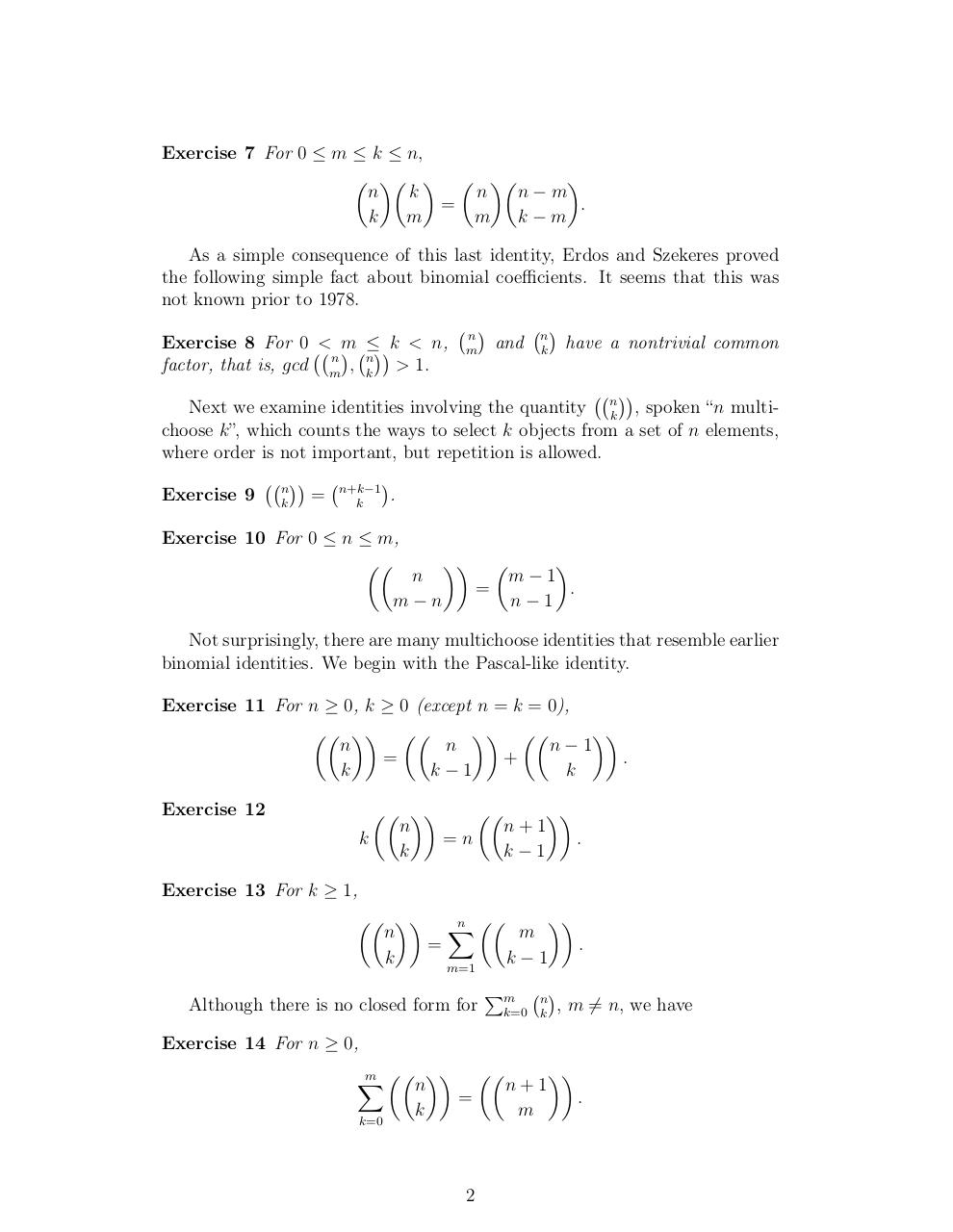# Day 10 Binomial coefficient identities .pdf

### File information

Original filename: Day 10-Binomial coefficient identities.pdf

This PDF 1.4 document has been generated by TeX / pdfTeX-1.40.3, and has been sent on pdf-archive.com on 24/01/2014 at 06:06, from IP address 169.231.x.x. The current document download page has been viewed 1236 times.
File size: 89 KB (2 pages).
Privacy: public file

Day 10-Binomial coefficient identities.pdf (PDF, 89 KB)

### Document preview

Combinatorial Analysis
Binomial coefficients identities

The binomial coefficient nk counts the number of k-subsets of a set of
n elements. These numbers have many fascinating properties and satisfy a
number of interesting identities. Recall that
  

n
n
=
.
k
n−k
Next we prove some more identities. Use combinatorial proofs.
Exercise 1 Show that, for all n and k positive integers such that 0 ≤ k ≤ n
(except n = k = 0)
  
 

n
n−1
n−1
=
+
.
k
k
k−1
This identity is called Pascal’s formula.
Exercise 2 For n ≥ 1,
X n 
k≥0

2k

= 2n−1 .

Exercise 3 Show that, for all n and k positive integers such that 0 ≤ k ≤ n,
 


n
n−1
k
=n
.
k
k−1
Exercise 4 For n ≥ 1,
 
n
X
n
k
= n2n−1 .
k
k=0
Exercise 5 Dividing both sides of the last identity by 2n allows us to give a
different combinatorial proof of the equivalent identity:

Pn
n
n
k=0 k k
= .
n
2
2
The next identity is called Vandermonde’s identity.
Exercise 6 For m ≥ 0, n ≥ 0,

 X

k  
m+n
m
n
=
.
k
j
k−j
j=0
The next identity has an interesting application to number theory.

Exercise 7 For 0 ≤ m ≤ k ≤ n,
    

n
k
n
n−m
=
.
k
m
m k−m
As a simple consequence of this last identity, Erdos and Szekeres proved
the following simple fact about binomial coefficients. It seems that this was
not known prior to 1978.


n
Exercise 8 For 0 &lt; m ≤k &lt; n, m
and nk have a nontrivial common
n
factor, that is, gcd m
, nk &gt; 1.

Next we examine identities involving the quantity nk , spoken “n multichoose k”, which counts the ways to select k objects from a set of n elements,
where order is not important, but repetition is allowed.


Exercise 9 nk = n+k−1
.
k
Exercise 10 For 0 ≤ n ≤ m,


n
m−n





m−1
=
.
n−1

Not surprisingly, there are many multichoose identities that resemble earlier
binomial identities. We begin with the Pascal-like identity.
Exercise 11 For n ≥ 0, k ≥ 0 (except n = k = 0),
  
 

n
n
n−1
=
+
.
k
k−1
k
Exercise 12

 


n
n+1
k
=n
.
k
k−1

Exercise 13 For k ≥ 1,
  X

n 
n
m
=
.
k
k

1
m=1
Although there is no closed form for

Pm

k=0

n
k



, m 6= n, we have

Exercise 14 For n ≥ 0,
m  
X
n
k=0

k


=

2

n+1
m


.Use the permanent link to the download page to share your document on Facebook, Twitter, LinkedIn, or directly with a contact by e-Mail, Messenger, Whatsapp, Line..

Use the short link to share your document on Twitter or by text message (SMS)

#### HTML Code

Copy the following HTML code to share your document on a Website or Blog

#### QR Code### Related keywords